# Dose effect analysis in Excel tutorial

2018-02-23

This tutorial will show you how to set up and interpret a dose effect logistic model in Excel using the XLSTAT add-on statistical software.

## Dose effect analysis

Probit, Logit and related modeling methods, are very useful techniques when one wants to understand or to predict the effect of a series of variables on a binary response variable (a variable which can take only two values, 0/1 or Yes/No, for example). Probit and Logit regression can be helpful to model the effect of doses in medicine, agriculture, or chemistry.

With the Dose effect analysis tool of XLSTAT you can either run the analysis on raw data (the response is given as 0s and 1s) or on aggregated data (the response is a sum of "successes" or ones, and the number of repetitions must also be available).

The methodology of logistic regression aims at modeling the probability of success depending on the values of the explanatory variables, which can be numerical or categorical variables.

## Dataset for dose effect analysis

The example treated here is an agro-chemical case where a phytosanitary product is tested at different doses on a given specie of caterpillars (grouped in boxes). The experimenters have recorded the initial number of caterpillars and the number of killed after 6 hours for the various doses. An experiment was conducted with a null dose to help evaluating the natural mortality effect.

An Excel sheet with both the data and the XLSTAT-Dose results can be downloaded by clicking here.

## Setting up a dose effect analysis

To activate the Dose Effects dialog box, start XLSTAT, then select the XLSTAT / Dose / Dose effect analysis command, or click on the coprresponding button of the Dose toolbar (see below).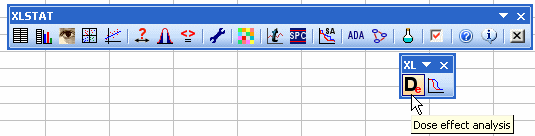When you click on the button, a dialog box appears.

Select the data on the Excel sheet. The Response variable corresponds to the column where the binary variable or the counts of positive cases are stored (NB: when using aggregated data the Observation weights must be selected). In this particular case we have one explanatory variable, the "Dose".

In the Options tab, we selected the Take the log option as we know that the Probit model is usually better fitted when the log of the dose is used instead of the dose itself.

The Probit model is one of the four possible models.

As we selected the column titles of all variables, we left checked the option Variable labels option.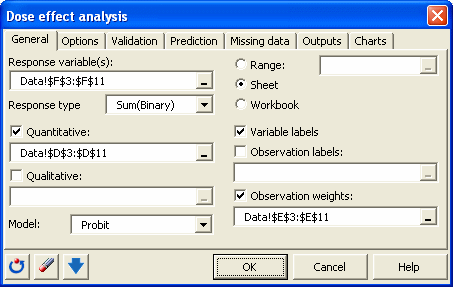In the Options tab, the Natural mortality parameter option was activated to take into account the natural mortality of the caterpillars.

We could either use a fixed (or user defined) value based on the null dose experiment (2/35 = 5.7 %), or ask XLSTAT to optimize the value. We chose to optimize it in this particular case.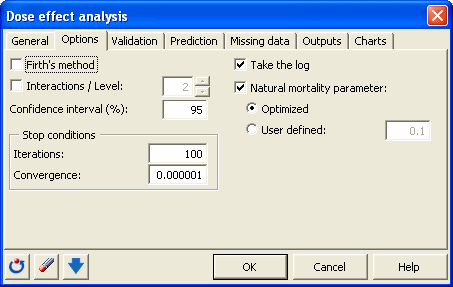The computations begin once you clicked the OK button. The results are displayed on a new sheet as requested in the dialog box.

## Interpreting the results of a dose effect analysis

The first table after the descriptive statistics gives several indicators of the quality of the model (or goodness of fit). These results are equivalent to the R2 and to the analysis of variance table in linear regression and ANOVA. The most important value to look at is the probability of Chi-square test on the log ratio of the likelihoods (-2Log(Loglike)). This is equivalent to the Fisher's F test: We try to evaluate if the variables bring significant information by comparing the model as it is defined with a simpler model with only one constant. In this case, as the probability is lower than 0.0001, we can conclude that significant information is brought by the Log(Dose) variable and the mortality.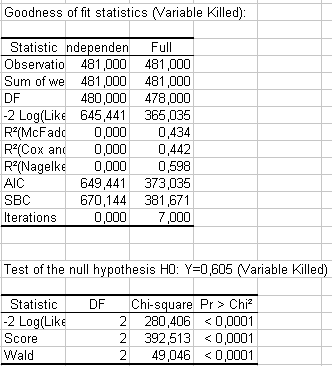The next table gives the estimates of the parameters of the model. We can see from the very low Chi-Square probabilitiy that the Log(Dose) variable explains well the variability of the mortality. The value of the natural mortality is also given. The optimized mortality is 0.126, meaning that, given the data, it is likely that 12.6% of the caterpillars died because of factors other than the dose. This is a higher than what the null dose experience gave (2/35 = 5.7 %).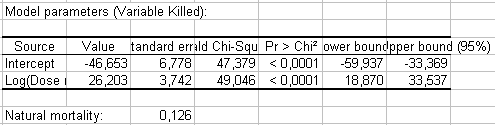A table gives the predicted values and the residuals. This table can be used to find some regions where the model doesn't fit well. The chart which is part of the results shows the data points, the model, and the confidence range around the model. The abscissa are displayed on a log scale if the "Take the log" was selected in the dialog box.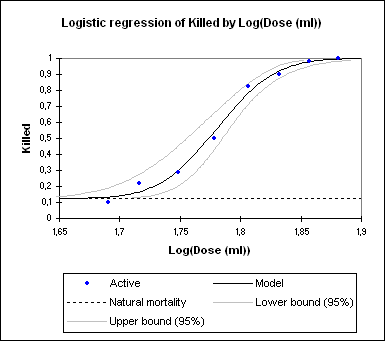When doing dose effects analysis you often compute the effective doses (EDs). They are used to answer the following question: which dose needs to be applied so that x% of the caterpillars are killed? The table below answers that question. In this case, the doses corresponding to the first 3 probabilities cannot be computed because they are below the natural mortality threshold (0.126).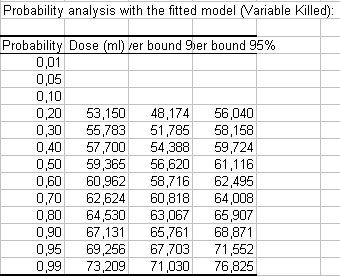The value corresponding to probability 0.5 is the EC50, the dose that kills 50% of the caterpillars. So here we have EC50 = 59.359.

#### Contact our technical support team: support@xlstat.com

https://cdn.desk.com/
false
desk